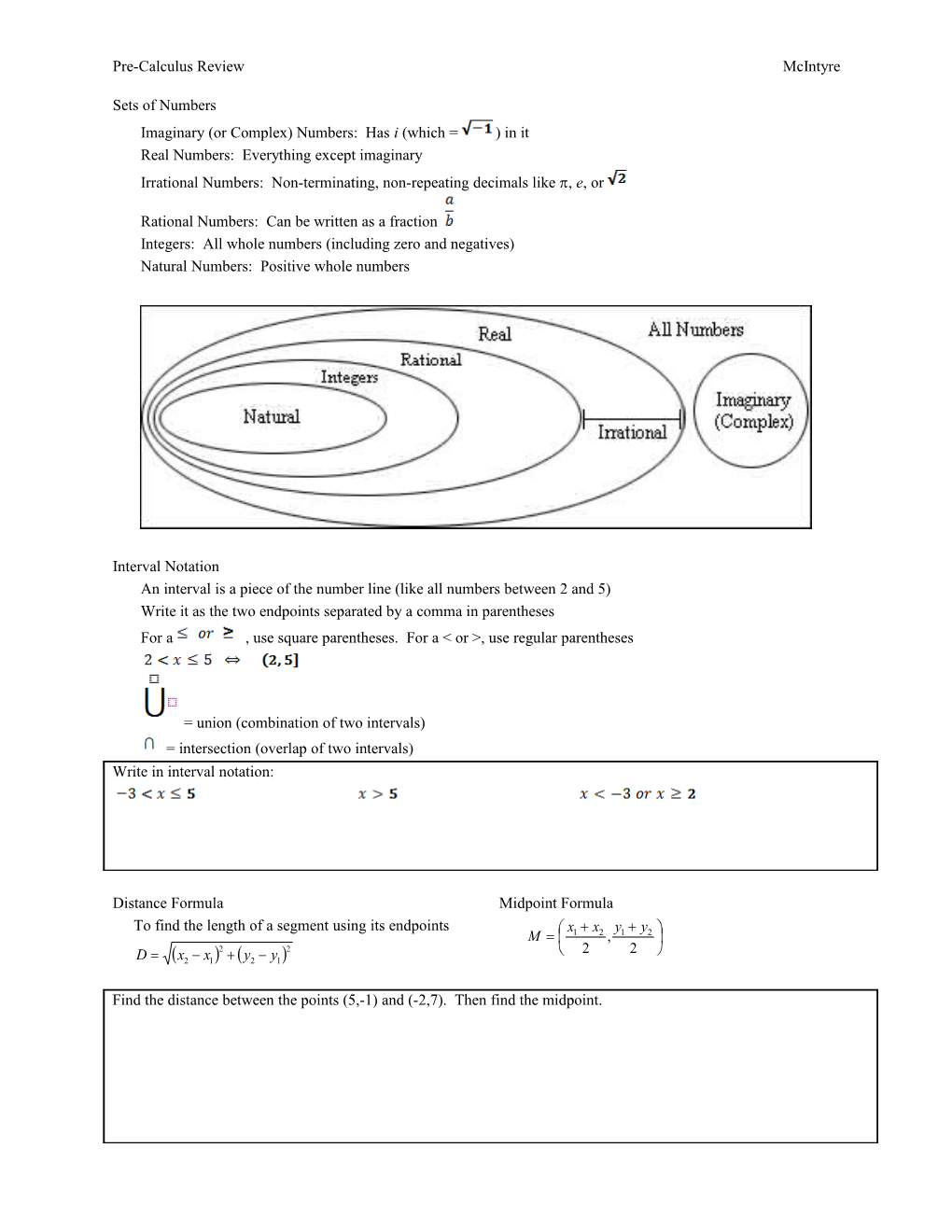# Real Numbers: Everything Except ImaginarySets of Numbers

Imaginary (or Complex) Numbers: Has i (which = ) in it

Real Numbers: Everything except imaginary

Irrational Numbers: Non-terminating, non-repeating decimals like , e, or

Rational Numbers: Can be written as a fraction

Integers: All whole numbers (including zero and negatives)

Natural Numbers: Positive whole numbersInterval Notation

An interval is a piece of the number line (like all numbers between 2 and 5)

Write it as the two endpoints separated by a comma in parentheses

For a , use square parentheses. For a < or >, use regular parentheses

= union (combination of two intervals)

= intersection (overlap of two intervals)

Write in interval notation:

Distance Formula
To find the length of a segment using its endpoints
/ Midpoint Formula

Find the distance between the points (5,-1) and (-2,7). Then find the midpoint.

Factoring

To solve an equation by factoring, it must be equal to zero!!!

Some common factoring patterns to know:

Solve for x: / Factor and simplify:

Slope

Lines

Slope-intercept form: y = mx + b

Point-Slope form: (y – y1) = m(x – x1)

Standard form: Ax + By = C (A, B and C are non-zero whole numbers)

Vertical line: x = constant

Horizontal line: y = constant

Parallel Lines: m1 = m2

Perpendicular Lines:

Find the equation of the line with a slope of -3 that passes through the point (-2, 5)

Intercepts

To find an intercept of any graph, set all other variables equal to zero and solve for the remaining variable

Find the x-intercept(s) of the graph of
Find the y-intercept

Functions

Function: An equation that relates 2 variables where each input has exactly one output (“vertical line test” says that if a vertical line crosses a graph more than once, then it is not a function)

Domain: Any x that can be put into the function and give a valid output (remember, the easiest way to find domain is you ask yourself what x can not be)

: All Real numbers =

: The empty set (nothing)

: “The set of all real numbers x, such that x is less than or equal to 5”

Range: All the values that f(x) takes on.

The easiest way to find range is using a graph. Look for a “floor” or “ceiling” the graph doesn’t pass

Find the domain of
Use a calculator to find the range
Find the domain of
Find the domain of

Function Notation: x is the input, and f(x) is the output (basically, f(x)is y)

For , find . (Note: this is called the difference quotient, and is important)

Restricting Domain

Sometimes you only want to look at a piece of a function. You can do this just by telling the reader what x can be.

For example:

Would be the right side of a parabola

Write an equation for the graph of the line segment that connects the points (2,5) and (-7,1).

Piecewise Functions

A function with more than one equation.

Each equation is used over a separate interval (just like restricting domain)

If

Absolute Value

|x| is the distance from zero to a number x on a number line (direction doesn’t matter)

Solve for x:
Express as a piecewise function

Graphing

/ x is the horizontal component (distance right or left)
f(x) is the vertical component (height) at x

Library of functions:

Transformations

shift up if C is positive, down if C is negative

shift left if C is positive, right if C is negative

stretch ↕ by a factor of C

stretch ↔ by a factor of C

* reflect and stretch before you shift!

Even Function: f(-x) = f(x) and the graph is symmetric about the y-axis

Odd Function: f(-x) = -f(x) and the graph is symmetric about the origin (180o rotation)

Note: cos is even, while sin and tan or odd. Reciprocals are the same.

Increasing: If x1 > x2, then f(x1) > f(x2) (“Rising” over a certain interval)

Decreasing: If x1 > x2, then f(x1) f(x2) (“Falling” over a certain interval)

Constant: For any x1, x2, f(x1) = f(x2) (“Flat” over a certain interval)

Is each function even, odd or neither?
/
Use the graph below to answer the questions.

/ 1. Does the graph represent a function?
2. If so, what is its domain?
3. If so, what is its range?
4. On what interval(s) is the graph increasing?
5. When x = -2, what is f(x)?
6. For what value(s) of x does f(x) = -3?
7. Draw f(x + 2) on the graph above

Compositions:

Note: To find the domain of f(g(x)) you must also consider the domain of g (the second function)

Find the domain of if and
Find the domain of

Inverses

* To find a functions inverse, switch x and y, and solve for the new y.

Notation:

Definition:

Graphs of inverse functions are reflections of each other in the line y = x

Important note:

Domain of = Range of

Range of = Domain of

If , find
If

Properties of Exponents

True or false:
Evaluate:

Logarithms

is read “log base 5 of x” and means “5 to what power equals x?”

b can be any positive number except 1

You can not take the log of a negative number or zero (domain is x > 0)

Logs and exponentials are inverses!

Properties of Logarithms (For any b > 0)

Converting logs to exponentials

Method 1 – Definition of inverses
Just switch x and y / Method 2 – Canceling out functions
Use property 3 or 4 above
Convert:
Find the domain of:

Solving Log/Exp Equations

Isolate the log/exp function

Switch forms using one of the methods above

Solve for x

Trigonometry

Know the unit circle!!!! I’m not going to re-re-re-re-teach it.

Know radian angles (2 = 360o)

Know Identities: Reciprocal, Pythagorean, Even/Odd

Evaluate:

/

Solving Trig Equations

Isolate the trig function(s) first (often done by factoring)

Solve using inverse trig (unit circle)